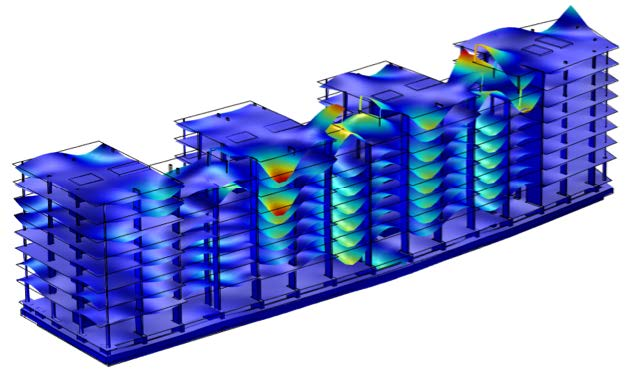Joe Bloggs

Chief of Testing

07799658747

# Numerical modelling

Solving engineering problems sometimes requires the use of computer predictions.  Our engineers are trained and experienced in the following modelling techniques:

• Finite element analysis (FE)
• Boundary element analysis (BE)
• Statistical energy analysis (SEA)
• Analytical models
• Empirical predictions
• Ray-tracing
• Computational fluid dynamics (CFD)Using these techniques requires access to specialist software; our engineers regularly use the following packages:

• MATLAB
• COMSOL Multiphysics
• ANSYS
• ACTRAN
• HyperMesh